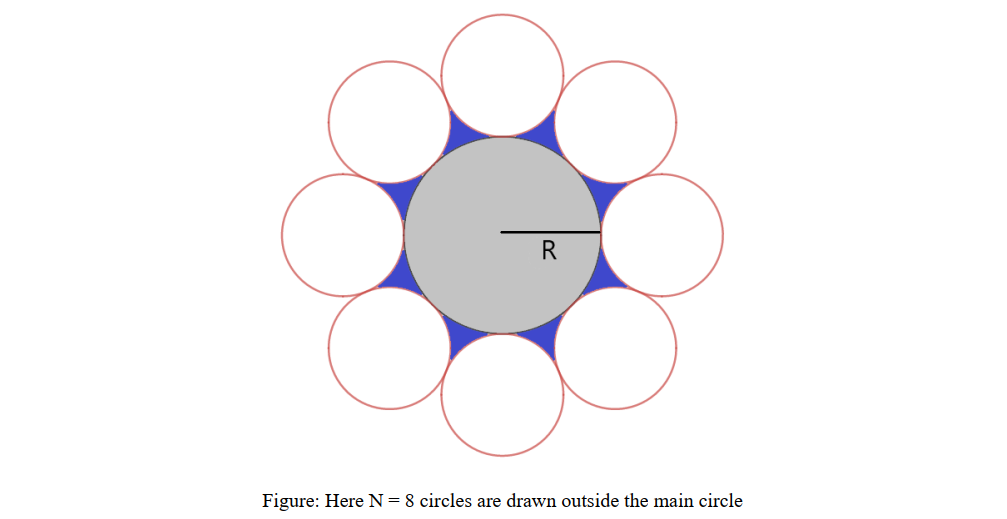# Practice on Toph

Participate in exhilarating programming contests, solve unique algorithm and data structure challenges and be a part of an awesome community.

# Quarantined With Circles

By Mehedi_Hassan · Limits 1s, 512 MB

You are given radius $R$ of a circle. Let’s call it “Main Circle”. You have to draw $N$ circles outside this main circle such that they follow the rules below $-$

(Compare with the picture below for better understanding)

1. Every outer circle touches the main circle.

2. Every outer circle touches exactly two more outer circles.

Find the area of the blue region.## Input

The first line contains an integer $T$ $\left(1 ≤ T ≤ 10^3\right)$, the number of test cases.

The next $T$ lines contains two integers, $R$ $\left(1 ≤ R ≤ 10^5\right)$ — radius of the main circle and $N$ $\left(3 ≤ N ≤ 10^5\right)$ — number of outer circles.

## Output

For each test case, print the area of the blue region.

Your answer is considered correct if its absolute or relative error doesn't exceed $10^{-6}$. Namely, if your answer is $a$, and the jury's answer is $b$, then your answer is accepted, if $\frac{|a-b|}{max(1, |b|)} \leq 10^{-6}$

## Sample

InputOutput
3
3 8
4 4
500 8

5.927964
29.785152
164665.680333


•Geometry

### Statistics

93% Solution Ratio

woolgathererEarliest, 1M ago

BigO_cutterFastest, 0.0s

woolgathererLightest, 131 kB

Hamim_CuetShortest, 447B

Login to submit

### Editorial

Here θ1=2π2n=πn.\theta_1 = \frac{2 \pi }{2n} = \frac{\pi }{n}.θ1​=2n2π​=nπ​. As OBOBOB is tangent t...

### Related Contests

 CUET Intra University Junior Contest (Round 1)Ended 1M ago Replay of CUET Intra University Junior Contest (Round 1)Ended 1M ago